# Boolean Operators

Knights and Knaves Riddle 1

### Knights and Knaves Riddle 1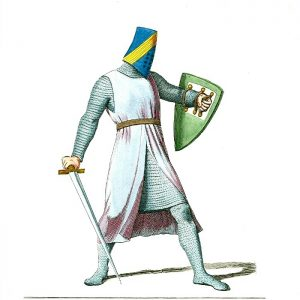You have been shipwrecked on the Knights and Knaves island. On this island there are only three types of people:

• Knights, who always tell the truth.
• Knaves, who always lie.
• Spies, who can either tell the truth or lie.

You are walking down a footpath and are approached by two people Amy and Bill.

• Bob says “At least one of use is a Knave.”
• You know that one is a knight and one is a knave.

Who is Amy and who is Bill?

Knights and Knaves Riddle 2

### Knights and Knaves Riddle 2

You are sunbathing on the beach when you are approached by three people.

You know that one is a knight, one is a knave and one is a spy. However, you don’t know who is who.

• Alfred says “Charlie is a Knave”
• Beatrix says “Alfred is a Knight”
• Charlie says “I am a spy”

Who is who?

AND Gates### AND Gates

Boolean AND gates are one most common logic gates used in programming. The work by returning True, only if both inputs are true.

### Truth Table

Input A Input B Output
FALSE FALSE FALSE
FALSE TRUE FALSE
TRUE FALSE FALSE
TRUE TRUE TRUE

Python AND gates

### Python AND gates

In python we can use and gates easily within our programs(in fact you probably already have!).

The example above is a pretty pointless example! Below are some more sensible uses of And gates…

Example – Password checker

In login systems both the username and password need to be correct in order for a user to be gained access.

Practice Activities

Practice 1

Write a python program that asks the users for..

Practice 2

Use comparison operators with and

OR Gates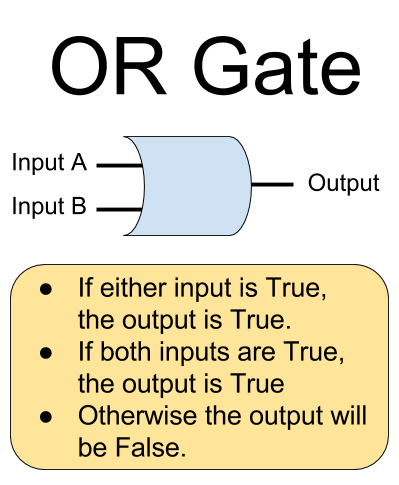### OR Gates

OR gates are another commonly used gate. They are less picky that AND gates – if either of the inputs is True (or both) then the gate will output True.

### OR Gate Truth Table

Input A Input B Output
FALSE FALSE FALSE
FALSE TRUE TRUE
TRUE FALSE TRUE
TRUE TRUE TRUE

Python or gates

Or gates are easy to use in Python. Here is a simple example.

Practice

### Practice 4

NOT Gates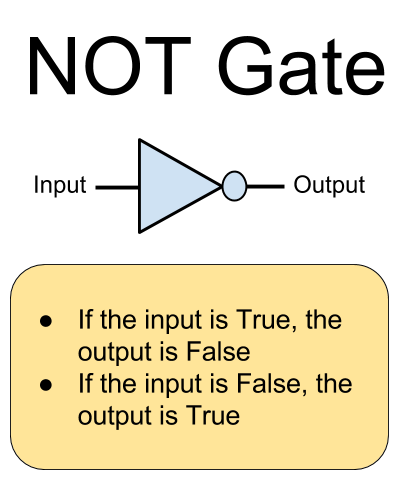### Not Gates

Not gates are the simplest form of gate. It’s job is simply to reserve whatever input it is given.

• If the input is True, the output is False.
• If the input is False, the output is True.

### Not gate Truth Table

Input A Output
FALSE TRUE
TRUE FALSE

Python NOT gates

Not gates are commonly used in Python code. Here is a simple example:

Practice 3
Practice 5

Practice 6

Combining Logic Gates

### Input 1 AND ( Input 2 OR Input 2)

As well as using Boolean Gates on their own you can also combine logic gates together. Order to work out how to solve combined logic gates you need to solve each of the left hand gates first, and then feed the outputs to the next gate along the line.

Example 1 - NOT with AND gate

#### Example 1  – NOT with AND gate (NAND gate)### Truth Table

Have a go at writing out the truth table for this combined gate.

Input 1 Input 2 Output
FALSE FALSE ??
FALSE TRUE ??
TRUE FALSE ??
TRUE TRUE ??
Answer
Input 1 Input 2 Output
FALSE FALSE TRUE
FALSE TRUE TRUE
TRUE FALSE TRUE
TRUE TRUE FALSE
Example 2 - Combined OR gates### Truth Table

Have a go at writing the truth table for this combined OR gate.

Input 1 Input 2 Input 3 Output
FALSE FALSE FALSE
FALSE FALSE TRUE
FALSE TRUE FALSE
FALSE TRUE TRUE
TRUE FALSE FALSE
TRUE FALSE TRUE
TRUE TRUE FALSE
TRUE TRUE TRUE
Answer
Input 1 Input 2 Input 3 Output
FALSE FALSE FALSE FALSE
FALSE FALSE TRUE TRUE
FALSE TRUE FALSE TRUE
FALSE TRUE TRUE TRUE
TRUE FALSE FALSE TRUE
TRUE FALSE TRUE TRUE
TRUE TRUE FALSE TRUE
TRUE TRUE TRUE TRUE
Combining gates using Python

### Combining gates in Python

If you ever need to combine logic gates in Python, the easiest way to do so is to use brackets. This makes writing and reading the gates much easier.

In the example above an AND gate is added, with the output feeding in to a NOT gate.

Practice - Python Questions

Take a look at these statements and work out whether they will output True or False!

Python Questions

1. True and True
2. True and False
3. True or True
4. False or False
5. False == True
6. False != False
7. not True
8. (True and True) and True
9. (True and False) and True
10. (False or False) or True
Practice - Logic Symbols

Logic Symbols

11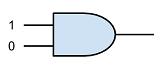1213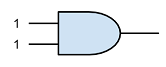14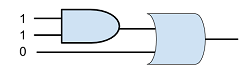15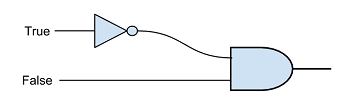http://learnpythonthehardway.org/book/ex28.html